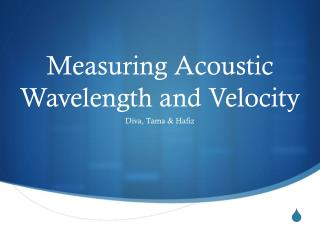# Measuring Acoustic Wavelength and Velocity - PowerPoint PPT PresentationDownload PresentationMeasuring Acoustic Wavelength and Velocity

Measuring Acoustic Wavelength and VelocityDownload Presentation## Measuring Acoustic Wavelength and Velocity

- - - - - - - - - - - - - - - - - - - - - - - - - - - E N D - - - - - - - - - - - - - - - - - - - - - - - - - - -
##### Presentation Transcript

1. Measuring Acoustic Wavelength and Velocity Diva, Tama & Hafiz

2. Introduction Wave properties: • Wavelength (λ): parallel displacement in one cycle. • Amplitude (a): maximum perpendicular displacement. • Period (t): time taken for one cycle. • Frequency (f): cycles undergone per unit time. • Velocity (v): linear displacement per unit time.

3. Resonance principle • Every half a cycle, a wave reaches its amplitude. • When the amplitude of a sound wave makes contact with a physical barrier (e.g. the inside of a tube), it amplifies the sound’s intensity. This effect is called resonance.

4. Hypothesis • The relationship between velocity, wavelength and frequency is defined as v = λf • By generating a sound wave with a constant frequency and finding its wavelength through the points of resonance, we might be able to find the speed of sound through a medium using a derivative of the above formula.

5. Materials • Biuret • Tuning forks (216 Hz, 288 Hz, 512 Hz) • Bucket • Retort stand

6. Procedure • Fill the biuret to the brim with tap water. • Tap the tuning fork on a hard surface and listen to its vibration as closely to the surface as possible. • Open the biuret and let the water flow out. When the water level reaches a point of resonance, the note should be momentarily amplified. • Rinse and repeat until no further resonance is heard.

7. Assuming that the sound wave experiences resonance every half a cycle, l2 – l1 = λ/2 • Where l = distance of a given resonance point from the surface.

8. Sources of error • Irregular water flow • External disturbances • Tuning fork vibration frequency not necessarily the same as the sound wave generated • Human error • False positives • Mistiming • Zero error/parallax

9. Results l2 – l1 = 2.1 cm, 3.5 cm Calculated length: 4.2 cm, 7.0 cm Mean result: 5.6 cm Calculated velocity: 12.096 ms-1 Average difference: 3.0 cm, 3.1 cm Avg. calculated length: 6.0 cm, 6.2 cm Mean result: 6.1 cm Calculated velocity: 13.176ms-1

10. l2 – l1 = 3.1 cm, 2.7 cm Calculated length: 6.2 cm, 5.4 cm Mean result: 5.8 cm Calculated velocity: 16.704 ms-1 Average difference: 3.7 cm, 3.1 cm Avg. calculated length: 7.4 cm, 6.2 cm Mean result: 6.8 cm Calculated velocity: 19.584 ms-1

11. l2 – l1 = 4.6 cm, 5.7 cm Calculated length: 9.2 cm, 11.4 cm Mean result: 10.3 cm Calculated velocity: 52.736ms-1 Average difference: 3.2 cm, 5.4 cm Avg. calculated length: 6.4 cm, 10.8 cm Mean result: 8.1 cm Calculated velocity: 41.472 ms-1

12. Observation • The values calculated were much lower than the known speed of sound in air (334.2 m/s) • This might be due to the experiment method, which depends on human hearing to take readings and is therefore prone to human error.

13. Conclusion • Though the concept is sound, a more reliable method of measurement is required to achieve proper results. • Hence, this lab session is inconclusive.

14. References • Brian Arnold et al. International A/AS-Level Physics. London: Hodder Education, 2008. • “Sound Waves”. Rice University Web Services. Rice University, n.d.